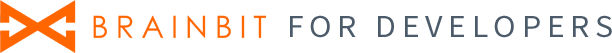# SpectrumPowerChannel

Package: com.neuromd.neurosdk

Spectrum power data channel. Heir to the base channel class BaseDoubleChannel. Spectrum power is calculated based on arrays of SpectrumChannel, channels specified in the constructor.

Includes the following fields:

 SpectrumPowerChannel Constructor Options: channels[]:Spectrum channel array SpectrumChannel.   lowFrequency: The lower limit of the frequency for calculating the spectrum power in Hz.   highFrequency: The upper limit of the frequency of the spectrum power calculation in Hz.   name: An arbitrary text channel identifier.   windowDuration: Spectrum calculation window size in seconds.   overlapCoeff: Calculation window overlap factor. Values range from 0 to 1, where 0 is no overlap and 1 is maximum overlap SpectrumPowerChannel Constructor. Calculation window overlap ratio: 0.95. Options: channels[]:Spectrum channel array SpectrumChannel.   lowFrequency: The lower limit of the frequency for calculating the spectrum power in Hz.   highFrequency: The upper limit of the frequency of the spectrum power calculation in Hz.   name: An arbitrary text channel identifier.   windowDuration: Spectrum calculation window size in seconds. SpectrumPowerChannel Constructor. Spectrum calculation window size: 8 seconds. Calculation window overlap factor: 0.95. Options: channels[]:Spectrum channel array SpectrumChannel.   lowFrequency: The lower limit of the frequency for calculating the spectrum power in Hz.   highFrequency: The upper limit of the frequency of the spectrum power calculation in Hz.   name: An arbitrary text channel identifier. SetWindowDuration Method for setting the size of the spectrum calculation window in seconds. SetOverlappingCoefficient Method of setting the coefficient of overlap of the calculation window. Values range from 0 to 1, where 0 is no overlap and 1 is maximum overlap SpectrumPower Static method for calculating spectrum power based on spectrum array. Options: lowFrequency: The lower limit of the frequency for calculating the spectrum power in Hz.   highFrequency: The upper limit of the frequency of the spectrum power calculation in Hz.   spectrum[]:Spectrum data array   frequencyStep: Spectrum calculation step in Hz.